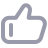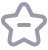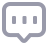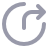# 外键缺少索引引发的死锁

355

### 问题描述

```SQL> CREATE TABLE t_p (id NUMBER PRIMARY KEY, name varchar2(30));
TABLE created.
SQL> CREATE TABLE t_f (fid NUMBER, f_name varchar2(30), FOREIGN KEY (fid) REFERENCES t_p);
TABLE created.
SQL> INSERT INTO t_p VALUES (1, 'a');
1 ROW created.
SQL> INSERT INTO t_f VALUES (1, 'a');
1 ROW created.
SQL> INSERT INTO t_p VALUES (2, 'b');
1 ROW created.
SQL> INSERT INTO t_f VALUES (2, 'c');
1 ROW created.
SQL> commit;
Commit complete.
SQL> DELETE t_f WHERE fid = 2;
1 ROW deleted.```

### 专家解答

```SQL2> DELETE t_f WHERE fid = 1;
1 ROW deleted.```

```SQL> DELETE t_p WHERE id = 2;

SQL2> DELETE t_p WHERE id = 1;```

```DELETE t_p WHERE id = 2
*
ERROR at line 1:
ORA-00060: deadlock detected while waiting FOR resource

SQL> ROLLBACK;
ROLLBACK complete.```

```1 ROW deleted.
SQL2> ROLLBACK;
ROLLBACK complete.
SQL2> CREATE INDEX ind_t_f_fid ON t_f(fid);
INDEX created.```

```SQL> DELETE t_f WHERE fid = 2;
1 ROW deleted.```

```SQL2> DELETE t_f WHERE fid = 1;
1 ROW deleted.```

```SQL> DELETE t_p WHERE id = 2;
1 ROW deleted.```

```SQL> DELETE t_p WHERE id = 1;
1 ROW deleted.```

「喜欢这篇文章，您的关注和赞赏是给作者最好的鼓励」

【版权声明】本文为墨天轮用户原创内容，转载时必须标注文章的来源（墨天轮），文章链接，文章作者等基本信息，否则作者和墨天轮有权追究责任。如果您发现墨天轮中有涉嫌抄袭或者侵权的内容，欢迎发送邮件至：contact@modb.pro进行举报，并提供相关证据，一经查实，墨天轮将立刻删除相关内容。